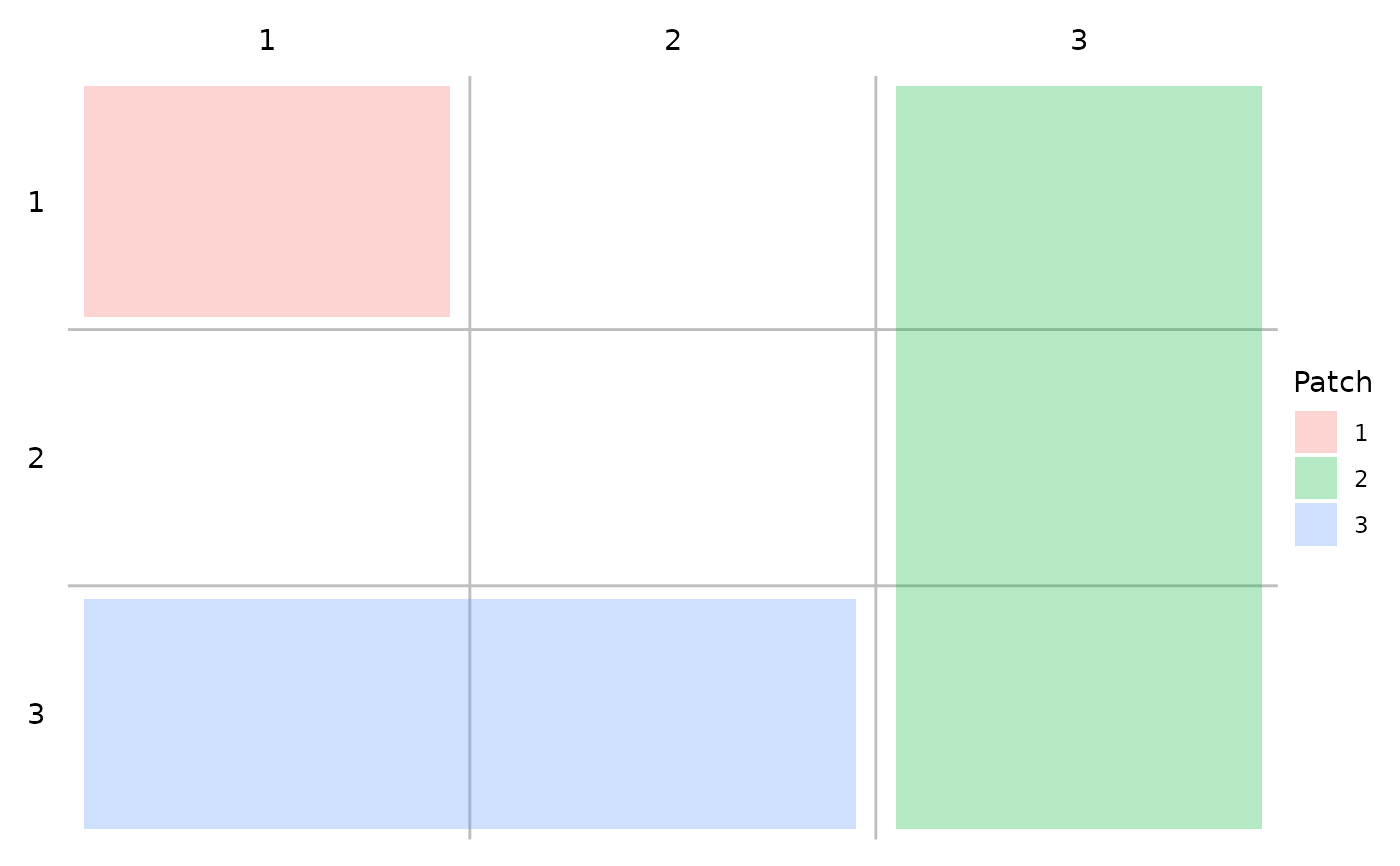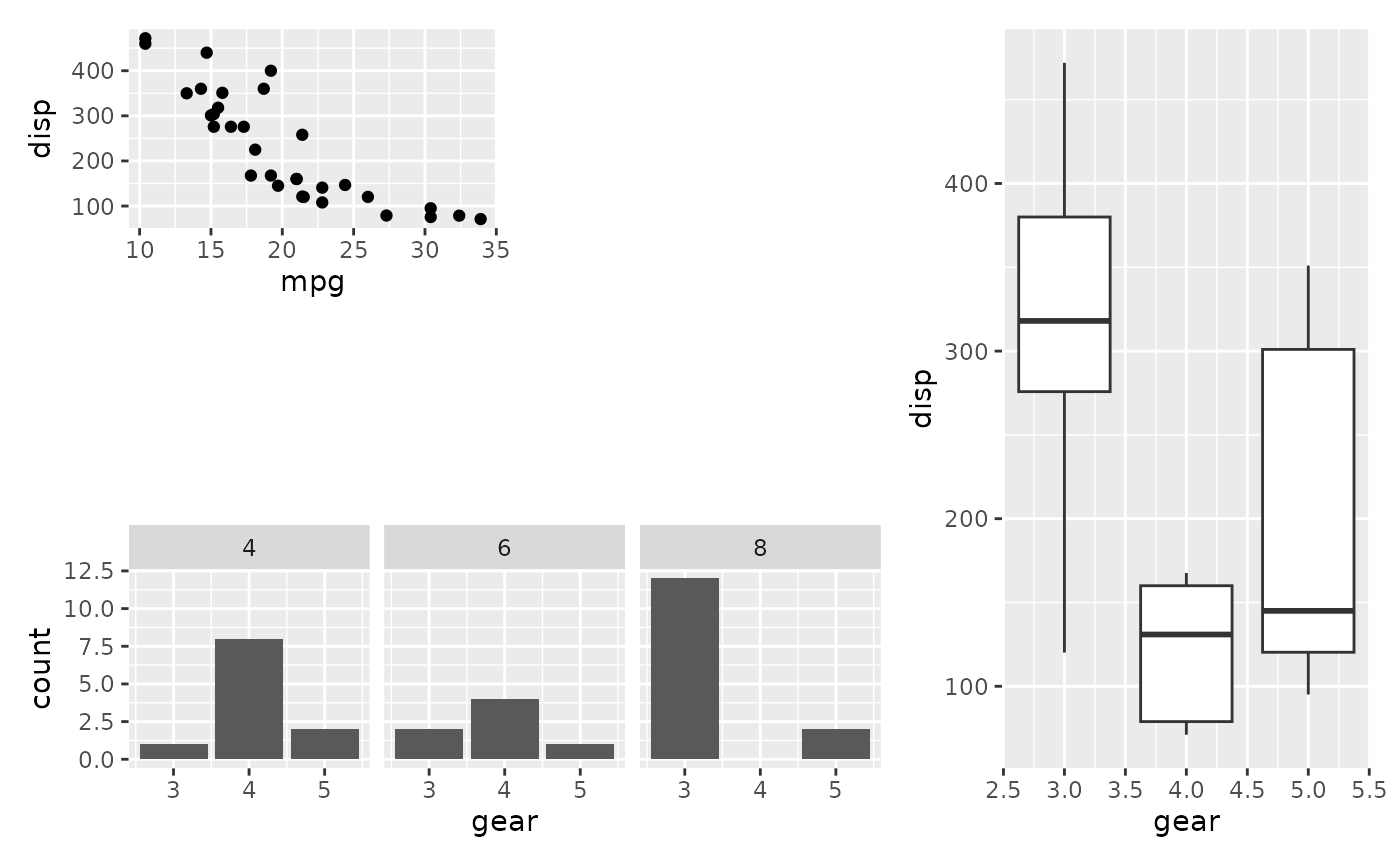This is a small helper used to specify a single area in a rectangular grid that should contain a plot. Objects constructed with area() can be concatenated together with c() in order to specify multiple areas.

area(t, l, b = t, r = l)

Arguments

t, b The top and bottom bounds of the area in the grid The left and right bounds of the area int the grid

Value

A patch_area object

Details

The grid that the areas are specified in reference to enumerate rows from top to bottom, and coloumns from left to right. This means that t and l should always be less or equal to b and r respectively. Instead of specifying area placement with a combination of area() calls, it is possible to instead pass in a single string

areas <- c(area(1, 1, 2, 1),
area(2, 3, 3, 3))

is equivalent to

areas < -"A##
A#B
##B"

For an example of this, see the plot_layout() examples.

Examples

library(ggplot2) p1 <- ggplot(mtcars) + geom_point(aes(mpg, disp)) p2 <- ggplot(mtcars) + geom_boxplot(aes(gear, disp, group = gear)) p3 <- ggplot(mtcars) + geom_bar(aes(gear)) + facet_wrap(~cyl) layout <- c( area(1, 1), area(1, 3, 3), area(3, 1, 3, 2) ) # Show the layout to make sure it looks as it should plot(layout)# Apply it to a patchwork p1 + p2 + p3 + plot_layout(design = layout)